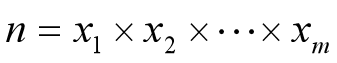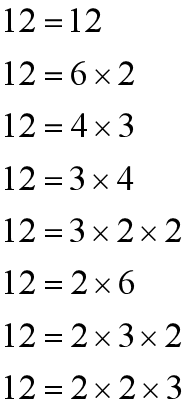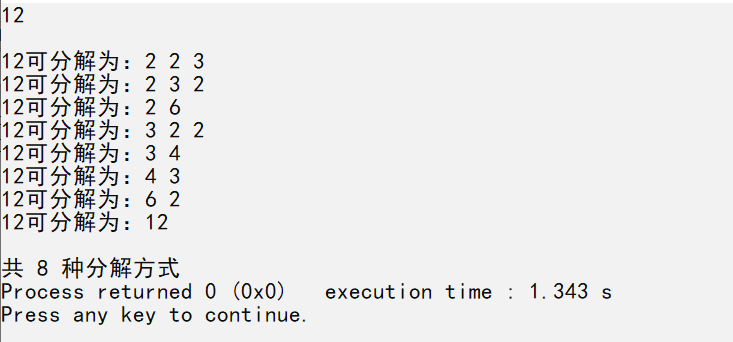• 2020-11-24 18:19:37

题目描述

输入一个不大于32767的正整数N，将它转换成一个二进制数

输入

输入只有一行，包括一个整数n(n<=32767)

输出

输出只有一行,包含一个二进制数。

样例输入

100

样例输出

1100100

要求

时间限制：1sec 内存限制：128M

#include <bits/stdc++.h>
using namespace std;
int b;
int main(){
int n;
cin>>n;
int c=0;
while(n!=0){  //将十进制转二进制
if(n%2==0){
b[c++] = 0;
}else{
b[c++] = 1;
}
n /= 2;
}
for(int i=c-1;i>=0;i--){//倒叙输出
cout<<b[i];
}
return 0;
}

更多相关内容
• 主要为大家详细介绍了C++实现正整数的四则运算表达式，文中示例代码介绍的非常详细，具有一定的参考价值，感兴趣的小伙伴们可以参考一下
• 大于1的正整数n可以分解为： 当n=12时，共有8种不同的分解式： 对于给定的正整数n，编程计算n共有多少种不同的分解式，并输出响应分解式子。 输入 数据有多行，给出正整数n（1≤n≤2000000000）。 输出 正整数n的...

## 题目

大于1的正整数n可以分解为：当n=12时，共有8种不同的分解式：对于给定的正整数n，编程计算n共有多少种不同的分解式，并输出响应分解式子。
输入
数据有多行，给出正整数n（1≤n≤2000000000）。
输出
正整数n的不同的分解式数量，以及分解式。

## 代码

#include <iostream>
#include <vector>
using namespace std;
vector <int> factor;
int total=0;
int num;
void output()
{
vector<int>::iterator v;
v = factor.begin();
while(v!=factor.end())
{
cout<<*v<<" ";
v++;
}
cout<<endl;
}
void solve(int n)
{
if(n == 1)
{
total++;
cout<<num<<"可分解为：";
output();//输出向量中的元素
}
else
for(int i = 2; i <= n; i++)
if (n % i == 0)
{
//如果i是n的因子，则将i压入栈
factor.push_back(i);
solve(n / i);
factor.pop_back();
}
}

int main()
{
cin>>num;
cout<<endl;
solve(num);
cout<<endl;
cout<<"共 "<<total<<" 种分解方式";
return 0;
}



## 运行展开全文• 编写程序实现两个超长正整数（每个最长80位数字）的减法运算。 【输入形式】 从键盘读入两个整数，要考虑输入高位可能为0的情况（如00083）。 1.第一行是超长正整数A； 2.第二行是超长正整数B； 【输出形式】 ...

【问题描述】
编写程序实现两个超长正整数（每个最长80位数字）的减法运算。

【输入形式】

从键盘读入两个整数，要考虑输入高位可能为0的情况（如00083）。
1. 第一行是超长正整数A；
2. 第二行是超长正整数B；

【输出形式】
输出只有一行，是长整数A减去长整数B的运算结果，从高到低依次输出各位数字。要求：若结果为0，则只输出一个0；否则输出的结果的最高位不能为0，并且各位数字紧密输出。
【输入样例】

234098
134098703578230056

【输出样例】
－134098703577995958

//超长正整数的减法
#include<stdio.h>
#include<string.h>
#include<iostream>
int c;
int judge(char a[], char b[])//判断前后位数
{
int i,j;
j=strlen(a);
if (strlen(a) < strlen(b))
{
return 0;
}
else if (strlen(a) > strlen(b))
{
return 1;
}
for (i = 0; i < j; i++)//此时两个数的位数相同
{
if (a[i] < b[i])
{
return 0;
}
}
return 1;
}//结果大于等于零返回1，结果小于零返回0
void minus(char a[], char b[], int k1, int k2)
{
int i, x, y;
x=strlen(a);
y=strlen(b);
char k;
for (i = 0; i<k1; i++, k1--)
{
k = a[i];
a[i] = a[k1];
a[k1] = k;
}
for (i = 0; i<k2; i++, k2--)
{
k = b[i];
b[i] = b[k2];
b[k2] = k;
}//讲两个数位数倒过来排序
for (i = 0; i<x; i++)
{
if (i >= y)
{
c[i] = a[i] - '0';
}//a比b的位数大时的位数不变
else
{
c[i] = a[i] - b[i];
}//a比b的位数小或相等时相减
}
for (i = 0; i<x; i++)
while (c[i]<0)
{
c[i] += 10;
c[i + 1] -= 1;
}//小于0时借1位
}
int main()
{
int i, j, k1, k2;
char a, b;
gets(a);
gets(b);
k1 = strlen(a) - 1;
k2 = strlen(b) - 1;
if (judge(a, b))//非零为true,零为false
{
minus(a, b, k1, k2);
}
else {
std::cout<<"-";
minus(b, a, k2, k1);
}
i = 99;
while (c[i] == 0 && i != 0)
{
i--;
}//计算c的位数
for (j = i; j >= 0; j--)
{
std::cout<<c[j];
}//倒着输出c
}

展开全文• ## C++ - 整数反转

万次阅读 多人点赞 2019-02-27 10:48:00
分享一个大牛的人工智能教程。零基础！通俗易懂！... * 题目很简单，完成函数reverse，实现把给定的一个整数反转。 * 举两个例子如下：x = 123，return 321；x = -123，return -321。 */ #in...

分享一个大牛的人工智能教程。零基础！通俗易懂！风趣幽默！希望你也加入到人工智能的队伍中来！请点击http://www.captainbed.net

/*
* Created by Chimomo
*
* 题目很简单，完成函数reverse，实现把给定的一个整数反转。
* 举两个例子如下：x = 123，return 321；x = -123，return -321。
*/

#include <iostream>

int reverse(int x) {

// The result integer to be returned.
int result = 0;

// The reminder.
int reminder;

// The sentinel to indicate positive or negative.
int negative = 0;

// Make negative number be positive and set sentinel.
if (x < 0) {
x = -x;
negative = 1;
}

// This loop does the "Inverse".
while (x % 10 >= 0 && x > 0) {
reminder = x % 10;
result = result * 10 + reminder;
x = x / 10;
}

// Restore to negative by sentinel.
if (negative == 1) {
result = -result;
}

return result;
}

int main() {
int num1 = 344;
int num2 = -90334;
printf("%d\r\n", reverse(num1));
printf("%d\r\n", reverse(num2));

return 0;
}

// Output:
/*
443
-43309

*/

展开全文• 主要介绍了C++初学者之根据输入的任何一个正整数，输出可能被表示的连续正整数的相关资料,需要的朋友可以参考下
• 求输入正整数的位数，逆序输出 知识点：if语句，while循环 //要求：给出一个不多于5位的正整数 //1）求出是几位数 2）分别打印出每一位数字 3）按逆序打印出各位数字，如原数123，输出321 #include<iostream> ...
• 输入一个不大于32767的整数n，将它转换成一个二进制数。 输入 输入只有一行，包括一个整数n(0<=n<=32767) 输出 输出只有一行。 样例输入 100 样例输出 1100100 程序代码 #include <bits/stdc++.h> ...进制转换
• 本文实例讲述了C++求四个正整数最大公约数的方法。分享给大家供大家参考，具体如下： /* * 作 者： 刘同宾 * 完成日期：2012 年 11 月 16 日 * 版 本 号：v1.0 * * 输入描述： 输入四个正整数，输出其最大公约数。 ...
• 题目 将一个正整数分解质因数。例如：输入90,输出90=233*5，输入5，输出5=5
• #include //设计算法，将某个大于1的数分成素因子的乘积 6=2*3 7=7 8=2*2*2 //1.判断当前数是不是素数，是直接返回 //2.否则，循环直到第一个它能整除的素数，当前数变为除以素数后的商，继续大循环。...
• 分享一个大牛的人工... * 求和为n的连续正整数序列 - C++ - by Chimomo * * 题目： 输入一个正整数n，输出所有和为n的连续正整数序列。例如：输入15，由于1+2+3+4+5=4+5+6=7+8=15，所以输出3个连续序列1-5、4-6...算法
• ## c++的整数表达

千次阅读 2017-12-29 16:32:33
后来发现是int表示范围不够的问题，sqrt(INT_MAX)的大小是46341，当i>46341的时候，int会表示不了这个好大的整数，然后就会溢出成一个负数，所以还是会小于n，会进入循环，所以访问passed[j]，就会出错。解决方法有...
• 将一个正整数分解质因数。例如：输入90,输出90=233*5，输入5，输出5=5 输入样例： 90 输出样例： 90=233*5 #include<iostream> using namespace std; int main() { int n,i; cin>>n; cout<<n&...
• js判断输入是否为正整数、浮点数等数字的函数代码JavaScript正则表达式校验非零的正整数实例jquery 正整数数字校验正则表达式JS只能输入正整数的...JavaScript正则表达式校验非正整数实例C++初学者之根据输入的任何一个正整数...
• k，但 n 能被 k 整除，则应打印出 k 的值，并用 n 除以 k 的商，作为新的整 数 n，重复执行第一步。  如果 n 不能被 k 整除，则用 k + 1 作为 k 的值，重复执行第一步。 #include <iostream> using ...c语言 c#
• ## C++整数类型

千次阅读 2016-12-21 20:31:08
C++中提供的整数类型有三种：int、long、short，每种类型又分为有符号和无符号两种类型。有符号整数既可以表示非负整数，又可以表示负整数；但是，无符号整数不能表示负数，只能表示非负整数。 （一)无符号整数  ...
• 下面小编就为大家带来一篇C++中几种将整数转换成二进制输出的方法总结。小编觉得挺不错的，现在就分享给大家，也给大家做个参考。一起跟随小编过来看看吧
• 输入：一个正整数 target 输出：所有和为 target 的连续正整数序列（至少含有两个数）。序列内的数字由小到大排列，不同序列按照首个数字从小到大排列。 思路分析：连续的正整数，可以采用暴力循环，也可以采用...算法 数据结构
• #include #include bool isValid(const std::string& str); int convert(const std::string& str); int main() { std::string str; std::cin >> str; if (isValid(str)) std::cout (str) << std字符串
• 设有 n 个正整数（n≤20，正整数在五位数以内），将它们连接成一排，组成一个最大的多位整数。 例如：n=3 时，3 个整数 13，312，343 联接成的最大整数为：34331213 又如：n=4 时，4 个整数 7，13，4，246 联接成的...
• C++之长整数C++之长整数C++之长整数C++之长整数C++之长整数C++之长整数C++之长整数C++之长整数C++之长整数C++之长整数C++之长整数C++之长整数C++之长整数C++之长整数C++之长整数C++之长整数C++之长整数
• (C/C++/Java默认的除法就是向0取整，python默认的是向负无穷取整。) 例如计算 100 * ( 2 + 12 ) - (20 / 3) * 2, 结果是1388。 输入 一个长度不超过100的字符串，代表要计算的算式。包含数字0-9以及+-*/()。 输入...
• while： #include<bits/stdc++.h> using namespace std; int main() { int a,x=0; cin>>a; while(a) { a/=10; x++; } cout<<x; return 0; } for： #include<... cin>.
• C++中提供的整数类型有三种：int、long、short，每种类型又分为有符号和无符号两种类型。有符号整数既可以表示非负整数，又可以表示负整数；但是，无符号整数不能表示负数，只能表示非负整数。 （一)无符号整数 ......

# c++正整数c++ 订阅